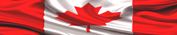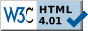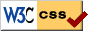HOME PAGE --------------- HELP+ » e-Design Notes --- » Switches, R, F » Resistors » Capacitors » Inductors » Diodes » Transistors --- » Potential Diff » Op Amps » Analog Filters --- » Boolean Algebra » Logic Gates » Flip-Flops » Logic ICs --- » Auto Injectors ---------------# ELECTRONIC DESIGN NOTES - OPERATIONAL AMPLIFIERSOperational Amplifier (Op Amp or OA for short) is an analog integrated circuit developed in 1965 by Mr. Robert Widlar at Fairchild Semiconductors Laboratories. Now, the beauty with this OA is, we work with it mathematically--well, almost mathematically.

In terms of construction, OAs are based on transistors' analog amplification [mostly FET transistors, but there are a few old OAs built with BJTs], and each OA contains tens or hundreds of transistors. However, one OA works as just one, functional, complex unit block, and it has many specific characteristics.

Working with analog signals is far from being easy, therefore OAs were designed to help us do the job. As hardware designers, the best thing is to avoid analog signals as much as it is possible. If you have an analog input, the first thing to do is, send it to an Analog-to-Decimal Conversion channel, and then use it as a decimal value inside controller's memory. However, that is not always possible. Although we live in a digital world today, there are two aspects which will forever require some analog processing: the inputs, and the outputs.

This Operational Amplifiers page is going to be a tough one to explain, because the topic is quite complex--though it is also extremely important, therefore it requires increased attention. The classic μA741 OA has been employed for this exemplification, and also for the simulation models used.

The following structure presents, summarily, a few general topics about working with Operational Amplifiers:

1. Operational Amplifier Specifications
2. Summing Amplifier
3. Noninverting Amplifier
4. Voltage Follower
5. Difference Amplifier
6. Integrator Amplifier
7. Differentiator Amplifier
8. Logarithmic Amplifier
9. Comparator Amplifier
10. Active Filters
11. Other Operational Amplifier Circuits

NOTE

The basic notions highlighted in this page are related to a few electronic design topics presented in the first part, Hardware Design, of
LEARN HARDWARE FIRMWARE AND SOFTWARE DESIGN.1. OPERATIONAL AMPLIFIER SPECIFICATIONS

The best thing to do is to download the datasheet (a PDF file) of μA741. O course, you will discover nothing impressive in there: just lists of specifications and a few graphs.

An OA has the following main characteristics:

1. High Voltage Gain, noted with Av, and having 103..109 levels of amplification.
2. High input impedance; the ideal case is (Zi --> ∞ ohms) [read this as: the input impedance tends to reach infinite values in ohms].
3. Wide Bandwidth.
4. Low output impedance; Zo --> 0 ohms.

Let's take a closer look at μA741 using a few common schematic models.

 μA741 MODELS Schematic Model DescriptionFig 1: μA741 pin names assignment Note that the OA has no direct connection to the board (circuit) ground. However, the circuits inside 741 are referenced to ground virtually, via external references, and you will see this aspect exemplified in the next pictures. On pins N2 and N1 it is (usually) wired a 10K potentiometer having the cursor connected to (V-). That potentiometer allows for input voltage and current offset adjustments.Fig 2: External (virtual) ground referenced model This model allows us to understand the following relations: Ii = (V1-V2) / Zi Vo = Av * (V1-V2) - Io* ZoFig 3: Inverted OA with "feedback"; model used to calculate OA's performances Model approximations: I2 = 0 (infinite input impedance) Vo = -Av * V2 (zero output impedance) Voltage Gain:  Av = -Vo / Vi = - (Zf / Zi) or Vi / Zi = - (Vo / Zo)

A few terms and formulas used to calculate OA schematics need to be explained.

 GENERAL OPERATIONAL AMPLIFIER  SPECIFICATION DATA AND FORMULAS Input Offset Voltage It happens some OAs do output little voltage, although there are no inputs. Therefore, we need to add a minor input offset voltage in order to have a perfect 0 V output. According to the datasheet, the Input Offset Voltage for μA741 is 1 mV, on average. Input Offset Current Normally, the two input currents should be equal when the output is 0 V. However, there is a slight imbalance. For μA741 it takes about 20 nA to compensate--which is negligible in most cases. Input Bias Current This is the DC current needed at the amplifier input terminal to establish the operation in the linear region. For the Inverted Feedback circuit presented above, the Input Bias Current Ib is:         Ib (appx.) = Vo / RfFig 4: Slew Rate         SR = dV / dT The "Slew Rate" is caused by the compensating capacitors (internal or external). For μA741 SR is 0.5 V/us (dT should be 10 us in Fig 4). ATTENTION [us] is read as "micro second"; "u" is a common notation in electronics instead of "μ" (micro) For sine-waves, SR allows us to calculate the maximum frequency of the OA:         fmax = SR / 2*PI*Vpp           Vpp being "the voltage peak to peak" Gain Bandwidth Product We have seen that the Slew Rate limits the maximum frequency. In addition, we have the capacitive reactance that limits the maximum frequency. Please remember the formula used to calculate capacitive reactance:          Xc = 1 / 2*PI*f*C          with f being the frequency and C the capacitance The higher is the frequency the smaller is Xc--as mentioned in previous Design Notes. In addition we have:          Av = (Rc || Xc) / Re   [Re comes from the input impedance Zi = 2*β*Re]          with Rc being the AC collector resistance of the OA input transistor          ||  means: in parallel with          Re is the AC emitter resistance of the OA input transistor Based on values particular to each OA type, the Av(f) graph is built, and it is named a "Baude diagram".          One decibel is defined as: 1 db = 20 log(Vo/Vi)--in this case log is in base 10 The decibel notation allows us to express Av in decibels when the frequency increases ten times,  fx = f * 10, meaning, Voltage Gain decreases ten times: Avx = Av / (-20 db) with x marking the new value. In other words, Voltage Gain (Av) is inversely proportional to the frequency by a factor of 10. When Av = 1 (at 0 db) the frequency limit is 1 MHz (for μA741). That 1 MHz value is named "Small-Signal Unity Gain Frequency (Ft)". However, sometimes another parameter is given in Data Sheets named "Transient Response Rise Time (Tr)", defined as the time it takes a waveform to raise from 10% to 90% of the final amplitude.          Unity Gain Frequency Bandwidth (BW) is calculated with:   BW = 0.35 / Tr          The Gain Bandwidth Product is: GBP = BW * Av                   with Av being the closed-loop (feedback) Voltage Gain of the OA circuit          BW = GBP / Av          BW = 1 MHz / 100 = 10 kHz for μA741 Power Supply and virtual ground As mentioned, the ground is virtual (related to) for (some) OAs, and this is an important concept because we relate all voltage and current formulas to that virtual ground. For μA741 we need two power supplies: one negative and one positive. The virtual ground is in between the two supplied voltages. Newer/different models require only one power supply, plus the ground connection. This facilitates working with OA, though the formulas used remain the same ATTENTION In all schematics/circuits presented here (V+) and (V-) pins of μA741 are not wired/connected, though they do need to be wired appropriately. First Approximation The difference in potential between the two input terminals of an OA is approximately zero. Exemplification:          Av = Vo / Vi for μA741 we have:          Vi = Vo / Av = 10 V /200000 = 50 uV (a negligible value) Second Approximation No current flows in or out the OA input terminals. Exemplification:          Ii = Vi / Zi for μA741 we have:          Ii = 50 uV / 2 MΩ = 25 pA (a negligible value)2. SUMMING AMPLIFIER

The summing OA circuit performs the mathematical operation of addition.

 SUMMING OPERATIONAL AMPLIFIERFig 5: Summing OA The Inverting Input pin (-) is also the summing node. The voltage at the summing node is 0 V. V1, V2, V3 drop all their voltages on R1, R2, R3 We calculate this circuit with the following formulas:         I1 + I2 + I3 = If         Vo = -Rf * (V1/R1 + V2/R2 + V3/R3) The summing OA schematic is used for DC and AC voltages/currents as well. Because each input voltage is dropped on its resistors, there is no signal mixing/distortion. The Averaging OA schematic is a particular case: each input resistors is equal to Ri, and Rf = Ri / N; N is the number of input resistors. Considering three inputs we have:           Vo = -Ri/3 * 1/Ri * (V1 + V2 + V3) = -(V1+V2+V3) / 33. NONINVERTING AMPLIFIER

You may have noticed that we can work with OAs as inverting or noninverting. We have seen the inverting circuit; time has come to look at the noninverting one.

 NONINVERTING OPERATIONAL AMPLIFIERFig 6: A noninverting OA We calculate this circuit with:         Ii = Vi / Ri   and   Vf = Ii * Rf         Vf = Vi * (Rf/Ri)         Vo = Vi + Vi * (Rf/Ri) = Vi * (1+ Rf/Ri)         Av = Vo/Vi = 1 + Rf/Ri There is no negative sign in the above Av formula. That means, there is no phase inversion and the Voltage Gain (Av) is never less than unity (1). The Input Impedance of the noninverting schematic is the impedance of the OA itself, which is very high.4. VOLTAGE FOLLOWER

 VOLTAGE FOLLOWER OPERATIONAL AMPLIFIERSFig 7: Voltage Follower This is a very simple circuit which duplicates the input DC or AC signals (with unity amplification). Due to the very high input impedance this circuit isolates the output. In addition, there is no phase shift, therefore the output replicates exactly the input. This is a safe input buffer circuit.5. DIFFERENCE AMPLIFIER

The difference amplifier is a combination of inverting and noninverting amplifiers.

 DIFFERENCE OPERATIONAL AMPLIFIERFig 8: A difference Amplifier         If   Ri = Ra = Rf = Rb                Vo = Vi1 + Vi2         If    Ri = Ra and Rf = Rb               Vo = (Rf/Ri) * (Vi2-Vi1) The Difference Amplifier circuit and the calculations presented above are used (mostly) in Instrumentation Amplifier schematics.6. INTEGRATOR AMPLIFIER

This is another mathematical function OAs perform. The schematics required are presented next.

 INTEGRATOR OPERATIONAL AMPLIFIERFig 9: Square-wave Integrator Red trace is Vi Blue trace is Vo The output is: Vo = (Vipp*Tdc) / (Ri*C) Tdc = duty cycle (50% in this case) Vipp = peak-to-peak input voltageFig 10: Sine-wave Integrator This circuit is perfectly similar to the one above. Only the input signal is changed to a sine-wave. Blue trace is Vi Red trace is Vo In this case the output is: Vo = Vipp / 2*PI*f*Ri*C Due to the added capacitance, we have a lagging phase shift of up to 90 degrees (PI/2)7. DIFFERENTIATOR AMPLIFIER

Mathematically, the differentiation is the reverse of integration. The following simulation graphs exemplify this operation.

 DIFFERENTIATOR OPERATIONAL AMPLIFIERFig 11: Sine-wave differentiation Red trace is Vi Blue trace is Vo Vopp=2*PI*F*Rf*C*IppFig 12: Square-wave differentiation Red trace is Vi Blue trace is Vo The derivation of the square-wave is obviousFig 13: Triangle-wave differentiation Red trace is Vi Blue trace is Vo Vopp= -Rf*C*(dVi/dt)8. LOGARITHMIC AMPLIFIER

For the analog logarithmic schematic, the output is proportional to the logarithm of the input signal. The antilogarithm performs the reverse of the logarithm. No simulation graphs are provided, because tuning these circuits (finding the right values for their components) is very difficult and a time consuming task (even as a simulation).

The good news is, there are a few ICs specifically designed to perform the log and antilog functions having all the needed/biasing components already built-in.

 LOGARITHMIC OPERATIONAL AMPLIFIERFig 14: Logarithmic amplifier This is a simplified schematic of the practical-implementation circuit.         Vo = K * log (Id/Iri)         K = proportional constant         Id = current through D         Iri = current through RiFig 15: Antilogarithmic amplifier This simplified schematic of the antilogarithm amplifier performs the inverse function of the above circuit9. COMPARATOR AMPLIFIER

Comparator amplifier circuits are used a lot in hardware design. Following is a schematic with feedback and memory (hysterensis). For practical implementations of comparator OA in electronic circuits please consult
LEARN HARDWARE FIRMWARE AND SOFTWARE DESIGN.

 COMPARATOR WITH HYSTERENSIS AMPLIFIERFig 16: Comparator with Feedback and  Hysterensis (Memory) Red trace is Vi Blue trace is Vo (transposed) This is a "zero crossing" type of comparator

10. ACTIVE FILTERS

This topic is presented in Design Notes 9 - Filters.11. OTHER OPERATIONAL AMPLIFIER CIRCUITS

There are very many types of OA schematics. A few of them are briefly listed next--this topic is way too vast to deal with in one Internet page.

1. Sine-wave oscillatorS
2. Square-wave oscillatorS
3. Voltage regulators
4. Voltage-to-current and current-to-voltage converters
5. Sample and hold circuits
7. Programmable Operational AmplifiersTake control of the extraordinary IT Revolution with Learn Hardware Firmware and Software Design--the easy way!» LINK TO "LOGICALLY STRUCTURED ENGLISH GRAMMAR"Page last updated on: July 25, 2018Site pages valid according to W3CStylesheets pages valid according to W3C